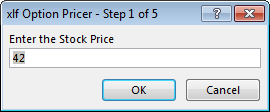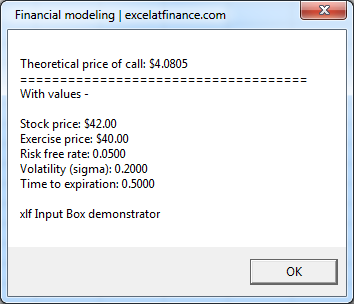Input box sequence

# VBA input box method

## A sequence of input boxes w/ the Black-Scholes model

There are five parameters to the Black-Scholes pricing model for an option on a non dividend paying stock. This module shows how a series of input boxes can be used to gather the values of these five parameters from the user. Assign the values to variables, then return the option price with a list of inputs to the user with a message box.

The input boxes use the VBA InputBox method, and are presented in the form of a wizard - each box is Step x of 5. Step 1 for the Stock price is shown in figure 1.Fig 1: The first input box - step 1 of the wizard, used to enter the stock price

The VBA code for the input box sequence is shown in code window 1.

## The VBA code

To allow easy testing of the box sequence, the user is able to allow default values in each box by setting the switch in line 9. TRUE displays default values, FALSE disables the default value feature. The CANCEL buttons exits the procedure.

Code 1: Macro BSCallOptBoxes displays a series of input boxes as a 5 step wizard. Allows optional default values - returns call price and parameters to a message box
Option Explicit
Option Base 1

'' ==============================================
Sub BSCallOptBoxes()
Dim Stock As Double, Exercise As Double, Rate As Double, Sigma As Double, Time As Double
Dim BSCallOpt As Double
Dim D(5) As String
Dim Switch As Boolean: Switch = True  '' <<<< Select: TRUE for defaults, FALSE for no defaults (blank)

If Switch Then D(1) = 42: D(2) = 40: D(3) = 0.05: D(4) = 0.2: D(5) = 0.5

Stock = Application.InputBox(Prompt:="Enter the Stock Price", _
Title:="xlf Option Pricer - Step 1 of 5", _
Default:=D(1), _
Type:=1)
If Stock = False Then Exit Sub
Exercise = Application.InputBox(Prompt:="Enter the Exercise Price", _
Title:="xlf Option Pricer - Step 2 of 5", _
Default:=D(2), _
Type:=1)
If Exercise = False Then Exit Sub
Rate = Application.InputBox(Prompt:="Enter the Risk Free rate as a decimal", _
Title:="xlf Option Pricer - Step 3 of 5", _
Default:=D(3), _
Type:=1)
If Rate = False Then Exit Sub
Sigma = Application.InputBox(Prompt:="Enter the Stock volatility as a decimal", _
Title:="xlf Option Pricer - Step 4 of 5", _
Default:=D(4), _
Type:=1)
If Sigma = False Then Exit Sub
Time = Application.InputBox(Prompt:="Enter the Time to Expiration, in years as a decimal", _
Title:="xlf Option Pricer - Step 5 of 5", _
Default:=D(5), _
Type:=1)
If Time = False Then Exit Sub

BSCallOpt = BSCall(Stock, Exercise, Rate, Sigma, Time)
MsgBox "Theoretical price of call: " & Format(BSCallOpt, "\$#,##0.0000") & vbNewLine _
& "====================================" & vbNewLine _
& "With values - " & vbNewLine & vbNewLine _
& "Stock price: " & Format(Stock, "Currency") & vbNewLine _
& "Exercise price: " & Format(Exercise, "Currency") & vbNewLine _
& "Risk free rate: " & Format(Rate, "0.0000") & vbNewLine _
& "Volatility (sigma): " & Format(Sigma, "0.0000") & vbNewLine _
& "Time to expiration: " & Format(Time, "0.0000") & vbNewLine & vbNewLine _
& "xlf Input Box demonstrator", , "  Financial modeling | excelatfinance.com"

End Sub


When the five parameters have been entered, the line 39 passes the values to the BSCall function procedure in code 2.

Code 2: Function BSCall displays a series of input boxes as a 5 step wizard. Allows optional default values - returns call price and parameters to a message box
Function BSCall(Stock As Double, Exercise As Double, Rate As Double, Sigma As Double, Time As Double) As Double
Dim d1 As Double, d2 As Double
With Application
d1 = (.Ln(Stock / Exercise) + (Rate + (Sigma ^ 2) / 2) * Time) / (Sigma * Sqr(Time))
d2 = (.Ln(Stock / Exercise) + (Rate - (Sigma ^ 2) / 2) * Time) / (Sigma * Sqr(Time))
BSCall = Stock * .Norm_S_Dist(d1, True) - Exercise * Exp(-Rate * Time) * .Norm_S_Dist(d2, True)
End With
End Function


Line 40 to 48 of code 1 then display the message box shown in figure 2.Fig 2: The final box in the sequence - displaying the Black-Scholes price and a list of parameter values